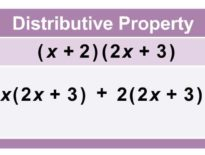# 6th-8th Grade Math Activity: Snail Population Sampling

Population sampling is a method used by statisticians to determine the total number of a population. Use the interactive activity below to see one way population is estimated. You can also recreate this activity on your own to determine the total number of a given population.

This interactive activity is embedded in our Math courses.

COURSE OVERVIEW

Students take a broader look at computational and problem-solving skills while learning the language of algebra. Students translate word phrases and sentences into mathematical expressions; analyze geometric figures; solve problems involving percentages, ratios, and proportions; graph different kinds of equations and inequalities; calculate statistical measures and probabilities; apply the Pythagorean theorem; and explain strategies for solving real-world problems. Online lessons provide demonstrations of key concepts, as well as interactive problems with contextual feedback. A textbook supplements the online material. Students who take Pre-Algebra are expected to have mastered the skills and concepts presented in the K12 Fundamentals of Geometry and Algebra course (or equivalent).

If you like this activity you can find an online school in your state.

Request a free catalog of K-12 Courses

Request the Scope & Sequence for a course

## 6th-8th Grade Math Activity: Snail Population Samp…